【ACL2020】一种新颖的成分句法树序列化方法

## 介绍

• 一是基于CKY的全局归一化方法，优化整棵句法树得分之和。也就是采用动态规划算法解析，时间复杂度较高（$O(n^3)$），但同时效果是目前SOTA的。
• 二是各种局部归一化方法，优化目标是单个目标得分（例如span、action、syntactic distance等等）。这一类方法具体包括基于shift-reduce的转移系统、各种序列化方法（例如syntactic distance）、基于CKY解码的局部归一化模型等，速度通常都很快，但由于局部归一化并没有考虑到全局特征，所以效果普遍较差。

## 序列化方法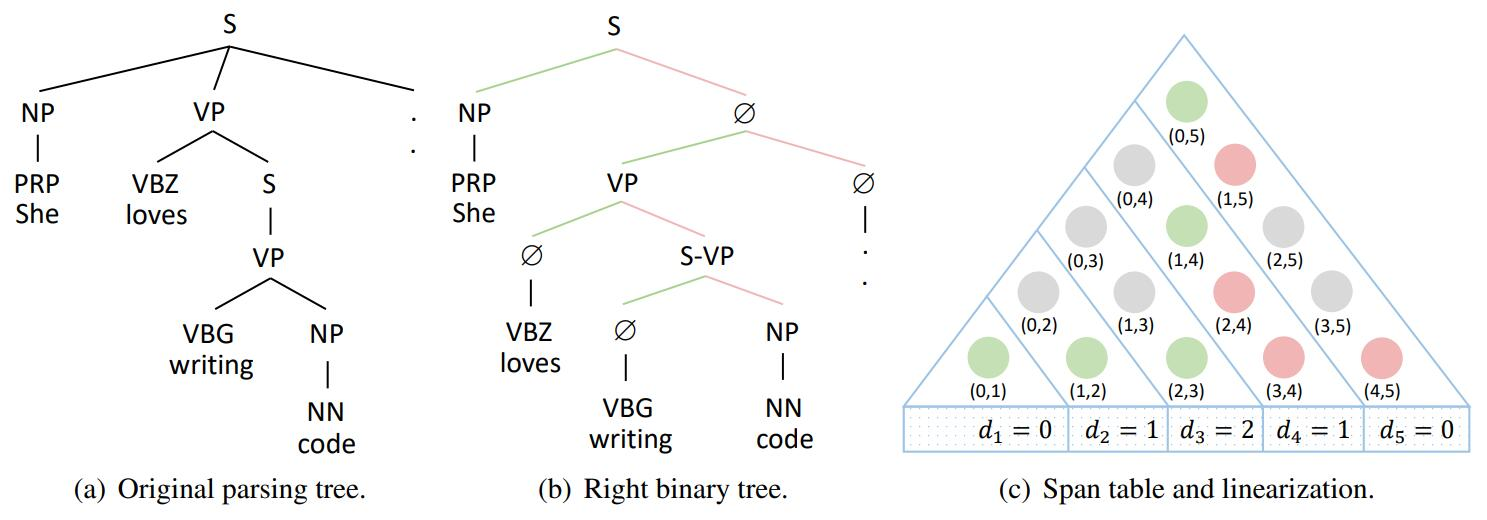• 一定满足 $d_i < i$ ，因为 $(d_i, i)$ 是某个左孩子。
• 对于任意的 $j > i$，$d_j$ 一定不会在 $(d_i, i)$ 范围里，否则的话 $(d_j, j)$ 就和 $(d_i, i)$产生交叉了。## 模型

$$x_i = [e_i; c_i; p_i].$$

$$l_i = \text{MLP}_l(h_i),\quad r_i = \text{MLP}_r(h_i).$$

$$\alpha_{ij} = l_i^\top {\bf W} r_j + b_1^\top l_i + b_2^\top r_j,$$

$$P(i|j) = \text{Softmax}_{i}(\alpha_{ij}), \forall i < j.$$

$$d_j = \mathop{\arg\max}_{i} {[P(i|j)]}, \forall i < j.$$

$$P(\ell|i, j) = \text{Softmax}(\text{MLP}_{\text{label}}([l_i; r_j]))_{\ell}.$$

$$\mathcal{L} = -\frac{1}{n} (\sum_{i = 1}^{n}{\log P(d_i | i)} + \sum_{(i, j, \ell) \in \mathcal{T}}{\log P(\ell|i, j)}).$$

### 反序列化

$$\mathcal{G}(i, j) = \max{\{P(i | k) \times \mathcal{G}(k, j) \mid i < k < j\}},$$

$$k \gets \max{\{k’ \mid d_{k’} \le i, i < k’ < j\}}.$$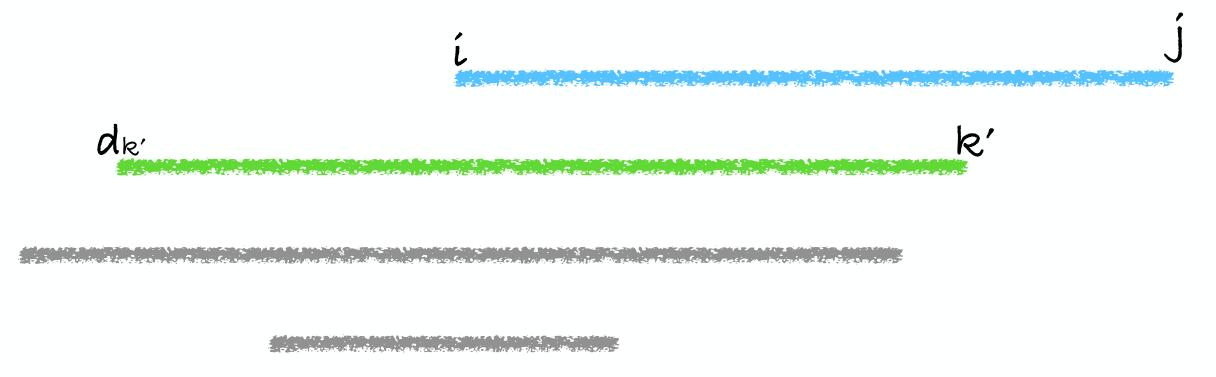$$k \gets \mathop{\arg\min}_{k’}[d_{k’}].$$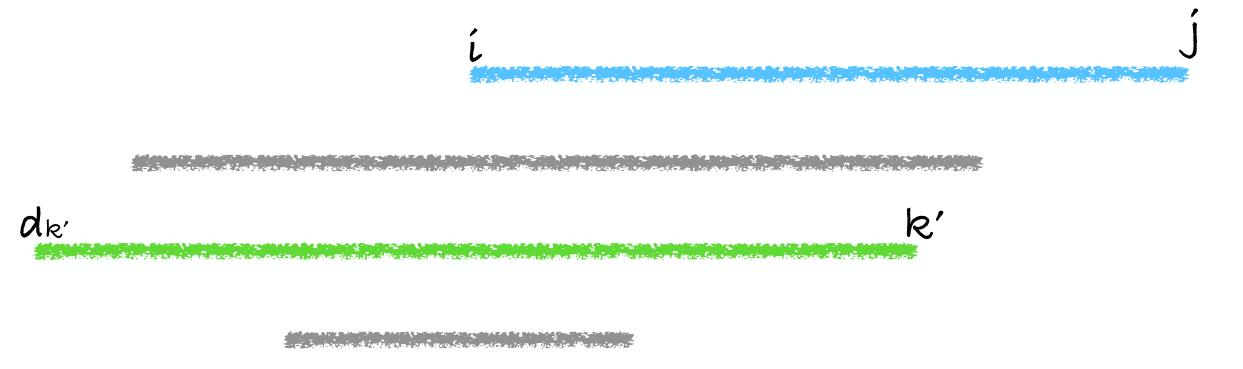## 实验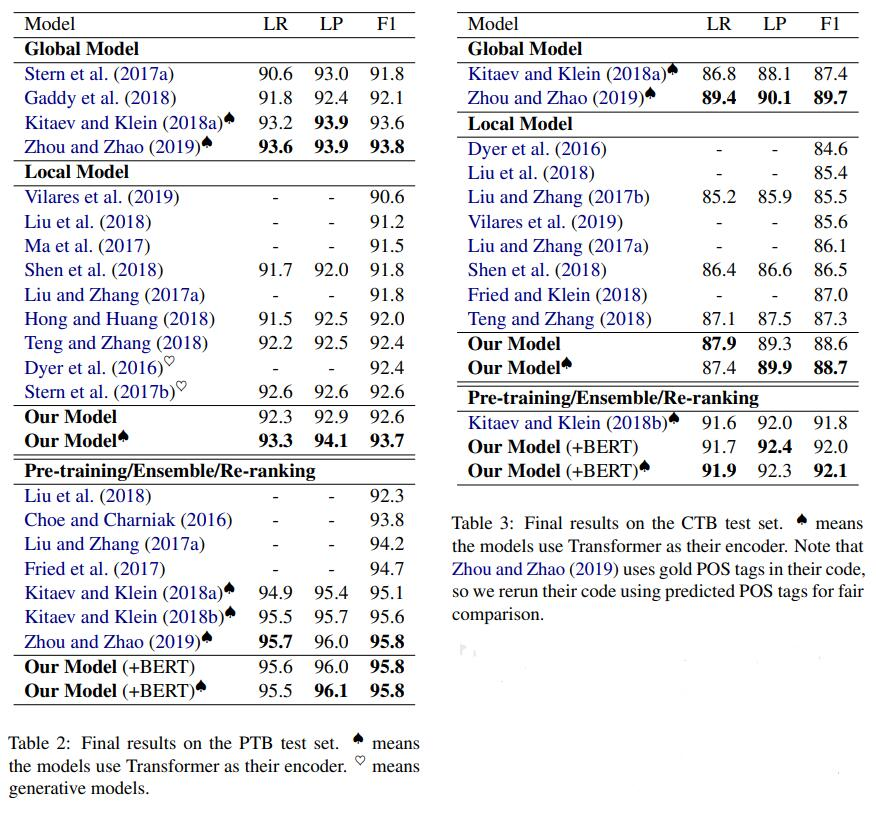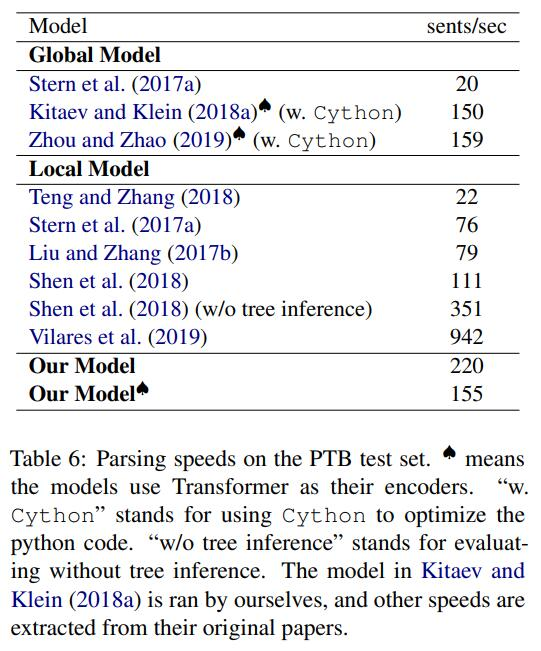## 一些思考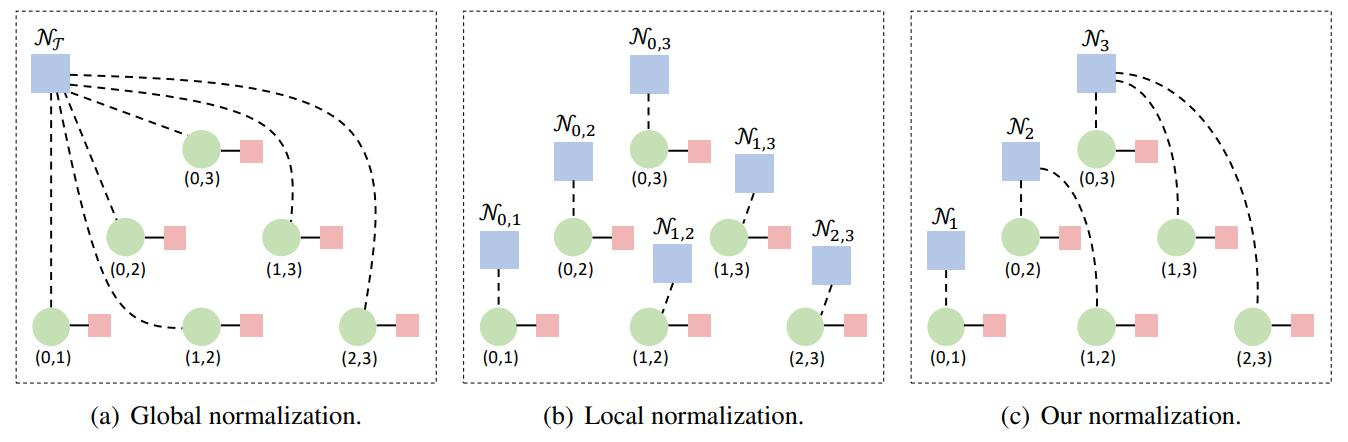• 利用我这个序列方法，GNN可以用上了。
• 如果用所有的右孩子，可以产生出另一套等价的序列表示，如何结合这两套表示来准确解码？
• 其他句法分析任务（CCG，semantic等）都可以转化为我的序列表示，如何联合学习这些任务？
• 利用这套表示，其他很多下游任务（机器翻译等）都可以加入句法信息，能否增强它们的性能？

## 尾声

转载规则

《【ACL2020】一种新颖的成分句法树序列化方法》 韦阳 采用 知识共享署名 4.0 国际许可协议 进行许可。
上一篇国庆碎碎念

2020-10-08【NLP 算法岗】提前批暑期实习面（试）经（历）

2020-04-26
目录